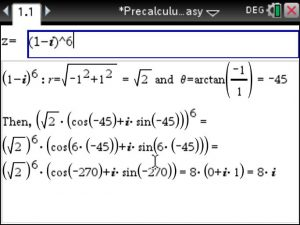## Equation App for the TiNspire CX

Taking Precalculus, Algebra 1 or Algebra 2 and need help with equations? Try our equation app! With no other app will you learn step by step how to solve and simplify equations in any math class you participate in! Take a look at our equation app video below and try our free trial of this equation app at www.tinspireapps.com

Posted on Categories algebra, preCalculus, steps, tinspirecx

## Precalculus Solver for the TiNspire CX

Need help with Precalculus homework? You’re in the right place! Our Precalculus solver app will be your best friend. This app will step by step solve your Precalculus problems immediately. Check out our Precalculus solver video below and try out the Precalculus trial at www.tinspireapps.com

Posted on Categories preCalculus, solver, tinspirecx

## DeMoivre Theorem using the TiNspire CX – PreCalculus Made Easy

Need to solve DeMoivre Theorem using the TiNspire CX Step by Step ?

Just enter a complex number in the format (a+bi)^n and lean back to observe the magic :PreCalculus Made Easy is the app to have when doing DeMoivre Formula – download it here https://www.tinspireapps.com/?a=pme

PS: Roots of Unity can be computed too using this app

Posted on Categories preCalculus, steps

## Step by Step PreCalculus app for the Ti-Nspire CAS CX available nowWatch this Youtube Video for an overview and examples at https://youtu.be/cRIs3oUicrw

Complete and Comprehensive.

Step by Step Solutions to : Functions (Polynomial, Exponential, Logarithmic and Trigonometric Functions), Trigonometry, Statistics, Discrete Mathematics, Algebra, Matrices, Complex Numbers, Sequences, Introductory Calculus.

Watch this Video on using the app doing PreCalculus homework at https://youtu.be/B9bYtG52XE4